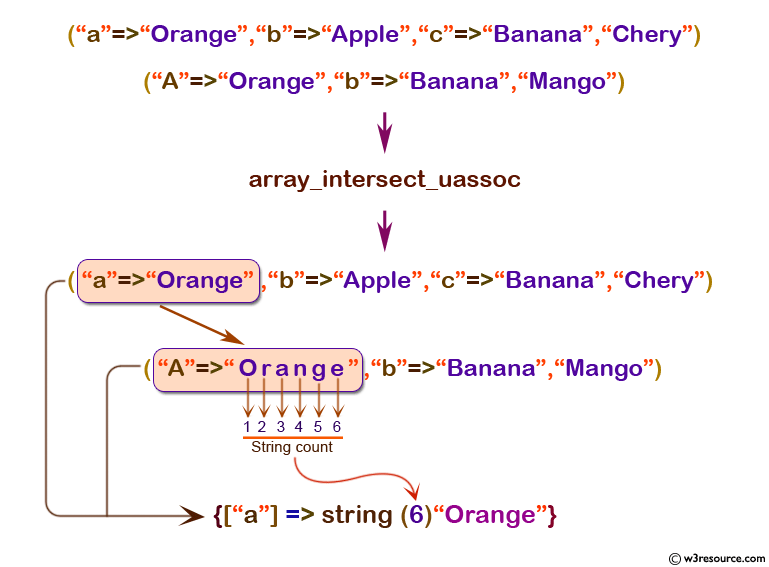# PHP: array_intersect_ uassoc() function

## PHP: Computes the intersection of arrays with additional index check, callback function

The array_intersect_uassoc() function is used to compare two or more arrays with an additional user supplied function (comparison function).

The comparison function returns an integer less than, equal to, or greater than zero if the first argument is considered to be respectively less than, equal to, or greater than the second.

Note: Before PHP 7.0.0 this integer had to be in the range from -2147483648 to 2147483647.

Version:

(PHP 4 and above)

Syntax:

`array_intersect_uassoc(array1, array2, array3 ..., user_function)`

Parameters:

Name Description Required /
Optional
Type
array1 The specified array which will be compared with other arrays. Required Array
array2 Compared with the first array. Required Array
array3 Compared with the first array. Optional
user_function A function to do the comparison. Required
-

Return value:

The values of array1 whose values exist in all of the arguments.

Value Type: Array

Example:

``````<?php
\$array1 = array("a" => "Orange", "b" => "Apple", "c" => "Banana", "Chery");
\$array2 = array("A" =>"Orange", "b" => "Banana","Mango");
\$result = var_dump(array_intersect_uassoc(\$array1, \$array2,"strcasecmp"));
print_r(\$result)
?>
``````

Output:

`array(1) {["a"]=> string(6) "Orange"}`

Pictorial Presentation:View the example in the browser

Practice here online :